﻿ "多维"多尺度重磁位场数据融合方法
 地球物理学报2021, Vol. 64Issue (4): 1453-1470PDF
"多维"多尺度重磁位场数据融合方法

1. 长安大学重磁方法技术研究所, 长安大学地质工程与测绘学院, 长安大学西部矿产资源与地质工程教育部重点实验室, 西安 710054;
2. 中国自然资源航空物探遥感中心, 北京 100083;
3. 自然资源部航空地球物理与遥感地质重点实验室, 北京 100083

The fusion with gravity and magnetic potential field data at multi-dimension and multi-scale
LIU FuXiang1, WANG WanYin1, JI XiaoLin1, XIONG ShengQing2,31. Institute of Gravity and Magnetic Technology, School of Geology Engineering and Geomatics, Chang'an University, Key Laboratory of Western China's Mineral Resources and Geological Engineering, Ministry of Education, Chang'an University, Xi'an 710054, China;
2. China Aero Geophysical Survey and Remote Sensing Center for Natural Resources, Beijing 100083, China;
3. Key Laboratory of Airborne Geophysics and Remote Sensing Geology, Ministry of Natural Resources, Beijing 100083, China
Abstract: With the application and development of multiple geophysical observation methods, the research on the fusion method of potential field data collected by different observation methods becomes more and more important. According to the different dimension and scales of observation, this paper will divide the data fusion into different dimension (single-dimension and multi-dimension) and scales (single-scale and multi-scale). In terms of the differences in the observation position and accuracy, and data benchmark of multi-dimension and multi-scale, the methods of iterative continuation method in spatial domain, weighted average and regression analysis are used to compute the potential field data measured by different observation methods to the same observation surface under the same standard. Based on this, a set of fusion method that is applied to potential field data at multi-dimension and multi-scale is proposed. The results of model testing and field data show that the fusion method works well in the fusion when the gravity and magnetic data at multi-dimension or multi-scale, following a small calculation error and consistency with the characteristics of potential field data. The fusion scheme at multi-dimension and multi-scale proposed in this paper is suitable for the potential field data of aviation, ground and marine, and has good practical significance and popularization value.
Keywords: Gravity anomaly    Magnetic anomaly    Data fusion    Multi-dimension and multi-scale
0 引言

1 多维多尺度重、磁位场数据融合方法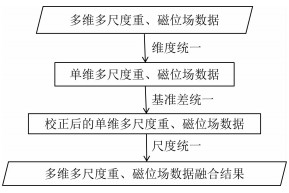图 1 多维多尺度重、磁位场数据融合计算流程示意图 Fig. 1 The schematic diagram of multi-dimension and multiscale gravity and magnetic potential data fusion calculation process
1.1 维度统一方法

(1) 位场向上延拓(1)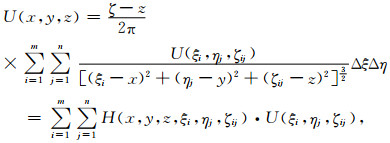(2)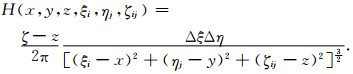(3)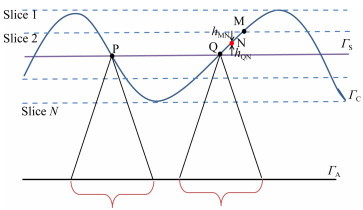图 2 向上延拓中滑动窗口和曲面切片示意图 Fig. 2 The schematic diagram of sliding window and surface slice in upward continuation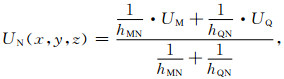(4)表 1 理论模型体空间位置坐标及物性参数 Table 1 The space position coordinates and physical property parameters of theoretical models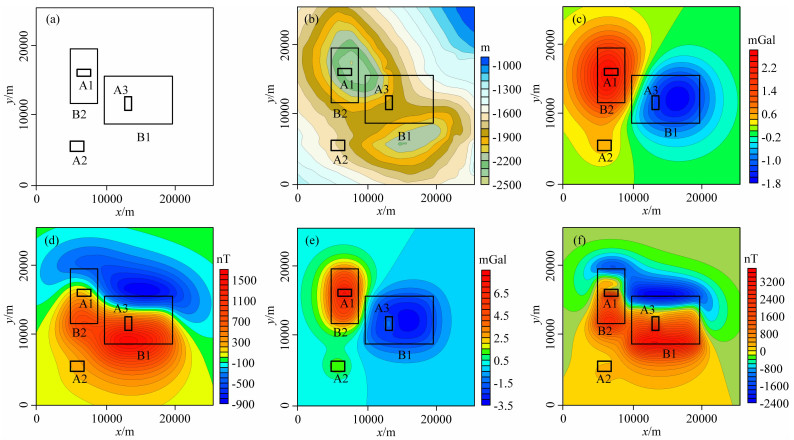图 3 理论模型平面位置、观测面一起伏及重、磁力异常等值线图 (a) 理论模型空间位置平面图；(b) 观测曲面一高程等值线图；(c) 模型体在观测曲面一上引起的重力异常等值线图；(d) 模型体在观测曲面一上引起的磁力异常等值线图；(e) 模型体在z=0 m平面上引起的重力异常等值线图；(f) 模型体在z=0 m平面上引起的磁力异常等值线图.图中黑色框线表示五个模型体的边界位置. Fig. 3 The plane position of theoretical models, observation surface One and its gravity and magnetic anomaly contour maps (a) The plane position of theoretical models; (b) The contour map of observation surface One elevation; (c) The contour map of gravity anomaly caused by the models on the observation surface One; (d) The contour map of magnetic anomaly caused by the models on the observation surface One; (e) The contour map of gravity anomaly caused by the models on the z=0 m plane; (f) The contour map of magnetic anomaly caused by the models on the z=0 m plane. The black solid lines represent the edge location of the five models.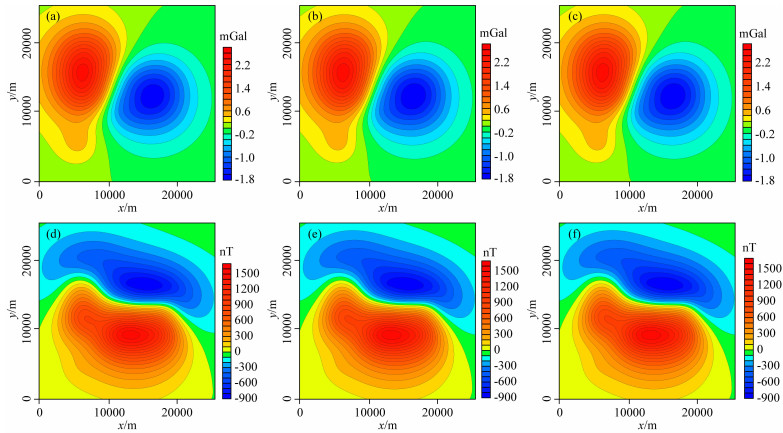图 4 理论模型向上延拓至观测曲面一重、磁力异常等值线图 (a) 无窗口延拓计算重力异常；(b) 加滑动窗口延拓计算重力异常；(c) 滑动窗口加曲面切片延拓计算重力异常；(d) 无窗口延拓计算磁力异常；(e) 加滑动窗口延拓计算磁力异常；(f) 滑动窗口加曲面切片延拓计算磁力异常. Fig. 4 The gravity and magnetic anomaly contour maps of theoretical models upward continuation calculation to observation surface One (a) The gravity anomaly of no-window upward continuation; (b) The gravity anomaly of slide-window upward continuation; (c) The gravity anomaly of slide-window and slice upward continuation; (d) The magnetic anomaly of no-window upward continuation; (e) The magnetic anomaly of slide-window upward continuation; (f) The magnetic anomaly of slide-window and slice upward continuation.表 2 理论模型向上延拓计算误差统计表 Table 2 The error table of upward continuation calculation of theoretical models

(2) 位场向下延拓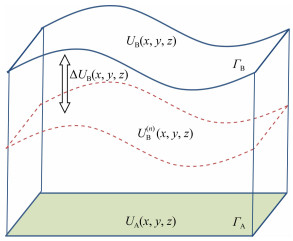图 5 积分-迭代法向下延拓示意图 Fig. 5 The schematic diagram of integral-iterative method for downward continuation

S1：将ΓB上的位场值赋给ΓA，作为其初值UA(1)(x, y, z)，即UA(1)(x, y, z)=UB(x, y, z)；

S2：ΓAΓB之间无场源，利用空间域向上延拓公式(式(1))，由ΓA初值UA(1)(x, y, z)计算ΓB上的位场值UB(1)(x, y, z)；

S3：计算UB(1)(x, y, z)与UA(1)(x, y, z)的偏差ΔUB(1)(x, y, z)，直接加入UA(x, y, z)中进行校正更新，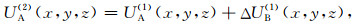(5)

S4:当UB(x, y, z)和UB(n)(x, y, z)的残差不满足精度要求时，重复S2步和S3步，得到迭代公式，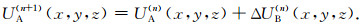(6)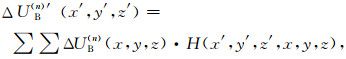(7)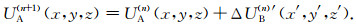(8)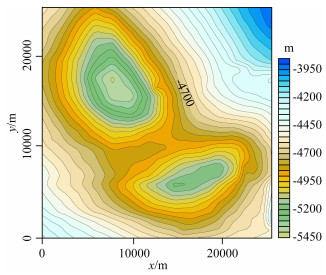图 6 观测曲面二地形起伏 Fig. 6 The contour map of observation surface Two elevation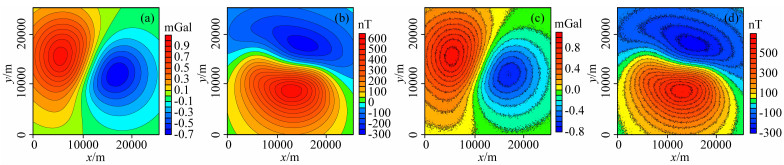图 7 理论模型观测曲面二重、磁力异常等值线图 (a) 重力异常；(b) 磁力异常；(c) 加噪声后的重力异常；(d) 加噪声后的磁力异常. Fig. 7 The contour maps of gravity and magnetic anomaly of theoretical models on the observation surface Two (a) The gravity anomaly; (b) The magnetic anomaly; (c) The gravity anomaly that added noise; (d) The magnetic anomaly that added noise.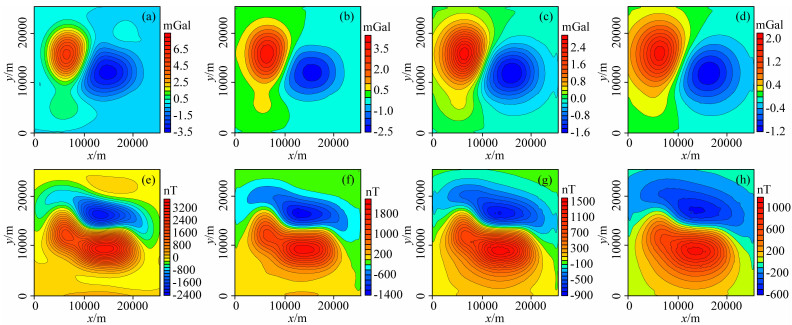图 8 观测曲面二向下延拓计算重、磁力异常等值线图 (a) z=0 m重力异常；(b) z=-1000 m重力异常；(c) z=-2000 m重力异常；(d) z=-3000 m重力异常；(e) z=0 m磁力异常；(f) z=-1000 m磁力异常；(g) z=-2000 m磁力异常；(h) z=-3000 m磁力异常. Fig. 8 The contour map of downward continuation results from observation surface Two (a) The gravity anomaly on the z=0 m plane; (b) The gravity anomaly on the z=-1000 m plane; (c) The gravity anomaly on the z=-2000 m plane; (d) The gravity anomaly on the z=-3000 m plane; (e) The magnetic anomaly on the z=0 m plane; (f) The magnetic anomaly on the z=-1000 m plane; (g) The magnetic anomaly on the z=-2000 m plane; (h) The magnetic anomaly on the z=-3000 m plane.表 3 理论模型重、磁力异常向下延拓计算误差统计表 Table 3 The error table of gravity or magnetic anomaly downward continuation calculation of theoretical models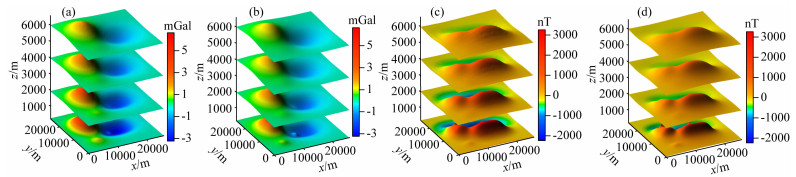图 9 多维数据融合计算重、磁力异常立体图 (a) 多维重力异常进行维度统一后的融合结果；(b) 多维重力异常当作单维直接进行处理的计算结果；(c) 多维磁力异常进行维度统一后的融合结果；(d) 多维磁力异常当作单维直接进行处理的计算结果. Fig. 9 The 3D surface map of multi-dimension gravity or magnetic anomalies fusion results (a) The fusion results of multi-dimension gravity anomalies after dimension unification; (b) The calculation results of multi-dimension gravity anomalies regarded as single-dimension data; (c) The fusion results of multi-dimension magnetic anomalies after dimension unification; (d) The calculation results of multi-dimension magnetic anomalies regarded as single-dimension data.表 4 理论模型多维重、磁力异常融合计算误差统计表 Table 4 The error table of multi-dimension gravity or magnetic anomaly fusion of theoretical models
1.2 基准差统一方法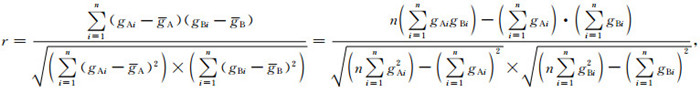(9)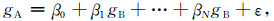(10)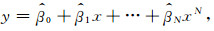(11)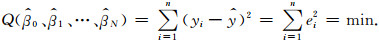(12)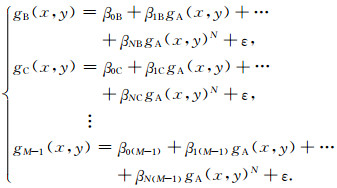(13)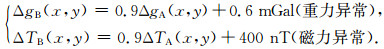(14)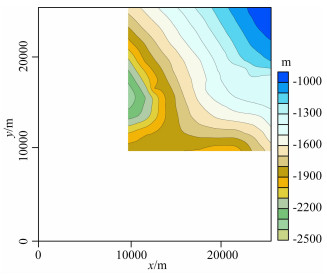图 10 观测曲面三地形起伏 Fig. 10 The contour map of observation surface Three elevation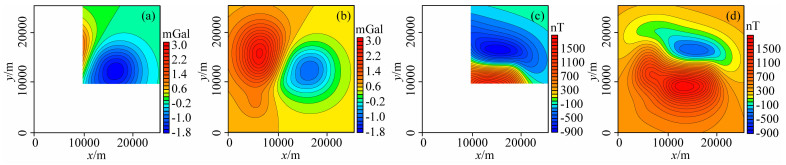图 11 单尺度原始重力、磁力数据等值线图 (a) 重力异常一；(b) 重力异常二；(c) 磁力异常一；(d) 磁力异常二.白色区域为数据空白区. Fig. 11 The contour map of original single-scale gravity and magnetic data (a) The first gravity anomaly; (b) The second gravity anomaly; (c) The first magnetic anomaly; (d) The second magnetic anomaly. The white areas indicate that there has no data in the areas.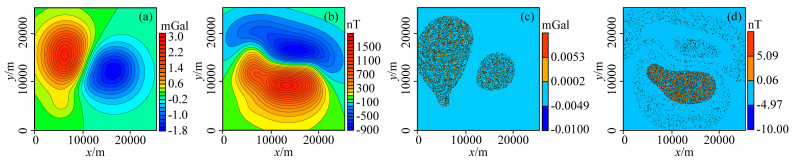图 12 单尺度重力、磁力异常回归分析计算结果及误差等值线图 (a) 重力异常回归分析结果；(b) 磁力异常回归分析结果；(c) 重力异常回归分析计算误差；(d) 磁力异常回归分析计算误差. Fig. 12 The contour map of regression results of single-scale gravity and magnetic anomalies (a) The regression results of gravity anomalies; (b) The regression results of magnetic anomalies; (c) The error of regression results of gravity anomalies; (d) The error of regression results of magnetic anomalies.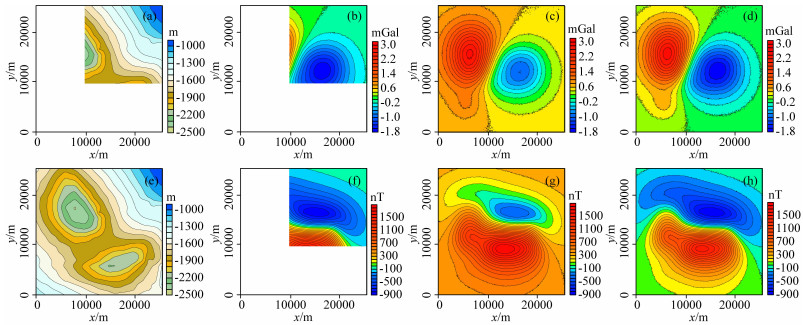图 13 多尺度观测面、原始数据及回归分析结果等值线图 (a) 观测面四；(b) 观测面四重力异常；(c) 观测面五重力异常；(d) 重力异常校正结果；(e) 观测面五；(f) 观测面四磁力异常；(g) 观测面五磁力异常；(h) 磁力异常校正结果.白色区域为数据空白区. Fig. 13 The contour map of multi-scale observation surface, raw data and regression result (a) The observation surface Four; (b) The gravity anomaly on observation surface Four; (c) The gravity anomaly on observation surface Five; (d) The regression results of gravity anomalies; (e) The observation surface Five; (f) The magnetic anomaly on observation surface Four; (g) The magnetic anomaly on observation surface Five; (h) The regression results of magnetic anomalies. The white areas indicate that there has no data in the areas.表 5 理论模型多尺度重、磁力异常回归分析校正误差统计表 Table 5 The error table of multi-scale gravity or magnetic anomaly regression of theoretical models
1.3 尺度统一方法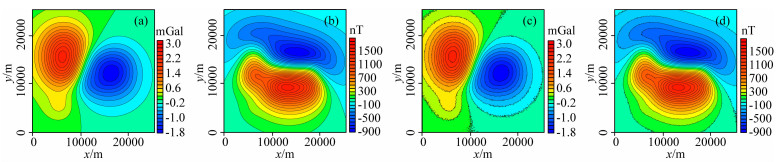图 14 单尺度和多尺度重、磁位场数据融合结果等值线图 (a) 单尺度重力异常融合结果；(b) 单尺度磁力异常融合结果；(c) 多尺度重力异常融合结果；(d) 多尺度磁力异常融合结果. Fig. 14 The contour map of fusion result of single-scale and multi-scale gravity and magnetic anomalies (a) The fusion result of single-scale gravity anomalies; (b) The fusion result of single-scale magnetic anomalies; (c) The fusion result of multi-scale gravity anomalies; (d) The fusion result of multi-scale magnetic anomalies.表 6 理论模型多尺度重、磁力异常融合计算误差统计表 Table 6 The error table of multi-scale gravity or magnetic anomaly fusion of theoretical models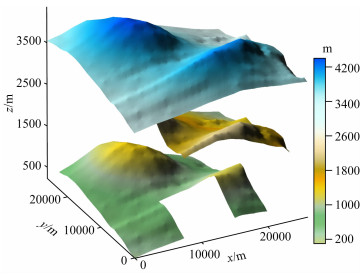图 15 多维多尺度原始观测面起伏 Fig. 15 The 3D surface map of original multidimension multi-scale observation surface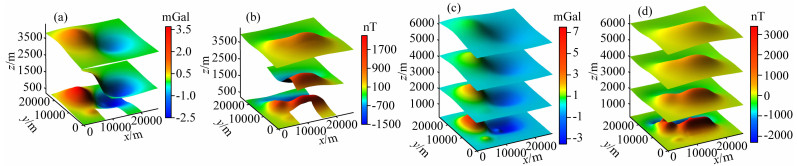图 16 多维多尺度重、磁异常及融合计算结果立体图 (a) 观测面重力异常；(b) 观测面磁力异常；(c) 重力异常融合结果；(d) 磁力异常融合结果. Fig. 16 The 3D surface map of multi-dimension multi-scale anomalies and fusion results of gravity and magnetic anomalies (a) The gravity anomalies on the observation surface; (b) The magnetic anomalies on the observation surface; (c) The fusion results of gravity anomalies; (d) The fusion results of magnetic anomalies.表 7 理论模型多维多尺度重、磁力异常融合误差统计表 Table 7 The error table of multi-dimension multi-scale gravity or magnetic anomaly fusion of theoretical models
2 实际资料处理试验

2.1 单维多尺度重力数据融合试验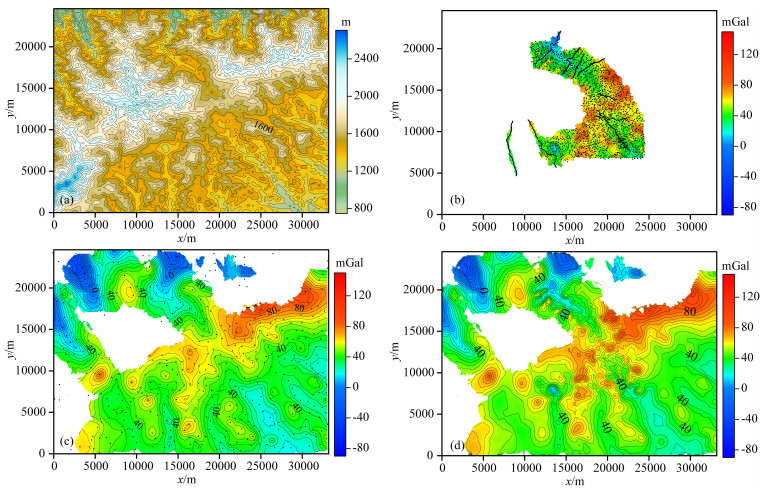图 17 地形起伏、观测重力异常及融合重力异常等值线图 (a) 地形起伏；(b) 数据体一1∶50000地面实测重力异常；(c) 数据体二1∶200000地面实测重力异常；(d) 单维多尺度融合重力异常；黑色点代表观测点. Fig. 17 The contour map of topography, measured gravity anomaly and the fusion result (a) The topography; (b) The gravity anomaly measured on the ground at scale 1 ∶ 50000; (c) The gravity anomaly measured on the ground at scale 1 ∶ 200000; (d) The fusion result of single-dimension multi-scale gravity anomaly; The black points represent the location of the observation points.

2.2 多维多尺度重力数据融合试验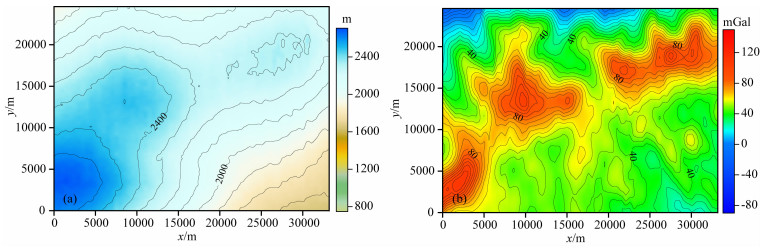图 18 航空观测GPS高度和实测重力异常等值线图 (a) 观测面GPS高度；(b) 1 ∶ 50000实测航空重力异常. Fig. 18 The contour map of observed surface GPS altitude and gravity anomaly measured by aviation (a) The GPS altitude of observation surface; (b) Measured gravity anomaly at scale 1 ∶ 50000 by aviation.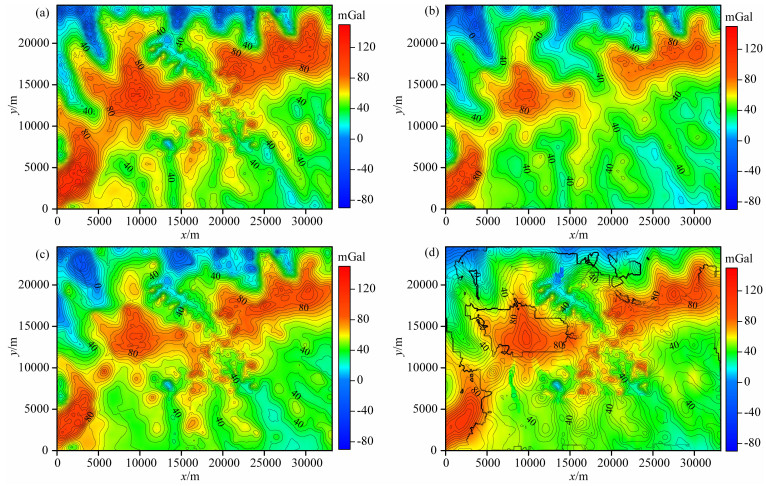图 19 多维多尺度融合重力异常等值线图 (a) 航空1∶50000与地面1∶50000重力异常融合；(b) 航空1∶50000与地面1∶200000重力异常融合；(c) 航空1∶50000与地面1∶200000、地面1∶50000重力异常融合；(d) 航空1∶50000与地面1∶200000、地面1∶50000重力异常当作单维直接处理结果. Fig. 19 The contour map of fusion results of multi-dimension multi-scale gravity anomaly (a) The fusion result of aviation 1 ∶ 50000 and ground 1 ∶ 50000 gravity anomaly; (b) The fusion result of aviation 1 ∶ 50000 and ground 1 ∶ 200000 gravity anomaly; (c) The fusion result of aviation 1 ∶ 50000, ground 1 ∶ 200000 and ground 1 ∶ 50000 gravity anomaly; (d) The processing result of aviation 1 ∶ 50000, ground 1 ∶ 200000 and ground 1 ∶ 50000 gravity anomaly regarded as singledimension data.

3 结论与建议

References